#### 期刊菜单

A Design Method of Tank Based on Winding Forming Process
DOI: 10.12677/JAST.2022.103007, PDF, HTML, XML, 下载: 68  浏览: 133  国家科技经费支持

Abstract: In order to improve the transport efficiency of carrier rocket and reduce the launching cost of rocket, the composite material storage tank instead of metal storage tank is the most direct and effective way. In this paper, a new design scheme of non-lined composite material storage tank is proposed. The design of the winding line and the winding layer is carried out based on the plane winding and the grid theory, and the winding scheme of the tank cylinder segment is put forward ultimately. Based on the classical laminated plate theory and Tsai-Wu failure criterion, single-angle strength checking is carried out to ensure the safety of the overall tank structure. The connecting structure of the upper and lower head pole hole and the design of the through hole are presented. By using ABAQUS, the solid model of the tank is established, and the finite element simulations of the winding layer, rubber layer, upper and lower flange connection structure of the tank under internal pressure are carried out. The feasibility and safety of the designed tank structure are discussed, and the improvement scheme for the existing problems in the design is proposed. The research work of this paper provides a theoretical basis for the forming of composite storage tanks.

1. 引言Figure 1. The Long March VFigure 2. NASA’s 5.5 m composite cryogenic storage tank

2. 贮箱结构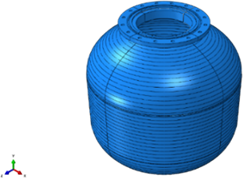(a)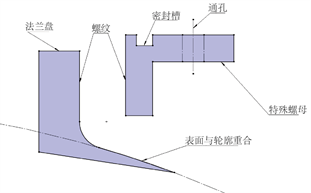(b)

Figure 3. Composite material storage tank. (a) Tank; (b) Connection structure

3. 贮箱设计

3.1. 缠绕层设计

$\mathrm{tan}\alpha =\frac{{y}_{1}+{y}_{2}}{{l}_{0}}=\frac{{r}_{1}+{r}_{2}}{l}$ (1)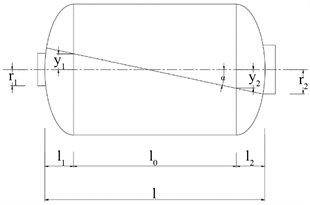Figure 4. Plane winding

$\begin{array}{l}{N}_{\phi }=\frac{1}{2}RP\\ {N}_{\theta }=RP\end{array}$ (2)

$\begin{array}{l}{t}_{f\alpha }=\frac{1}{2{\left(\mathrm{cos}\alpha \right)}^{2}}\cdot \frac{RP}{\left[{\sigma }_{f}\right]}\\ {t}_{f90}=\frac{2-{\left(\mathrm{tan}\alpha \right)}^{2}}{2}\cdot \frac{RP}{\left[{\sigma }_{f}\right]}\end{array}$ (3)

${t}_{f\alpha 实}={t}_{f\alpha }/k$ (4)

$\left(\begin{array}{c}{\epsilon }_{\phi }\\ {\epsilon }_{\theta }\end{array}\right)={\left[\begin{array}{cc}{A}_{11}& {A}_{12}\\ {A}_{21}& {A}_{22}\end{array}\right]}^{-1}\left(\begin{array}{c}{N}_{\phi }\\ {N}_{\phi }\end{array}\right)$ (5)

${A}_{ij}={\int }_{-h/2}^{h/2}{\stackrel{¯}{Q}}_{ij}^{\left(k\right)}\text{d}z=\underset{k=1}{\overset{n}{\sum }}{\stackrel{¯}{Q}}_{ij}^{\left(k\right)}\left({z}_{k}-{z}_{k-1}\right)=\underset{k=1}{\overset{n}{\sum }}{\stackrel{¯}{Q}}_{ij}^{\left(k\right)}{t}_{k}$ (6)

$\begin{array}{l}{\stackrel{¯}{Q}}_{11}={Q}_{11}{c}^{4}+2\left({Q}_{12}+2{Q}_{66}\right){c}^{2}{s}^{2}+{Q}_{22}{s}^{4}\\ {\stackrel{¯}{Q}}_{22}={Q}_{11}{s}^{4}+2\left({Q}_{12}+2{Q}_{66}\right){c}^{2}{s}^{2}+{Q}_{22}{c}^{4}\\ {\stackrel{¯}{Q}}_{12}=\left({Q}_{11}+{Q}_{12}-4{Q}_{66}\right){c}^{2}{s}^{2}+{Q}_{12}\left({c}^{4}+{s}^{4}\right)\\ {\stackrel{¯}{Q}}_{16}=\left({Q}_{11}-{Q}_{12}-2{Q}_{66}\right){c}^{3}s-\left({Q}_{22}-{Q}_{12}-2{Q}_{66}\right)c{s}^{3}\\ {\stackrel{¯}{Q}}_{26}=\left({Q}_{11}-{Q}_{12}-2{Q}_{66}\right)c{s}^{3}-\left({Q}_{22}-{Q}_{12}-2{Q}_{66}\right){c}^{3}s\\ {\stackrel{¯}{Q}}_{66}=\left({Q}_{11}+{Q}_{22}-2{Q}_{12}\right){c}^{2}{s}^{2}+{Q}_{66}{\left({c}^{2}-{s}^{2}\right)}^{2}\end{array}$ (7)

$\begin{array}{l}{Q}_{11}=\frac{{E}_{1}}{1-{\upsilon }_{12}{\upsilon }_{21}},{Q}_{22}=\frac{{E}_{2}}{1-{\upsilon }_{12}{\upsilon }_{21}}\\ {Q}_{12}=\frac{{\upsilon }_{12}{E}_{2}}{1-{\upsilon }_{12}{\upsilon }_{21}},{Q}_{66}={G}_{12}\end{array}$ (8)

$\left(\begin{array}{c}{\sigma }_{x}\\ {\sigma }_{y}\\ {\tau }_{xy}\end{array}\right)=\left(\begin{array}{ccc}{\stackrel{¯}{Q}}_{11}& {\stackrel{¯}{Q}}_{12}& {\stackrel{¯}{Q}}_{16}\\ {\stackrel{¯}{Q}}_{21}& {\stackrel{¯}{Q}}_{22}& {\stackrel{¯}{Q}}_{26}\\ {\stackrel{¯}{Q}}_{61}& {\stackrel{¯}{Q}}_{62}& {\stackrel{¯}{Q}}_{66}\end{array}\right)\left(\begin{array}{c}{\epsilon }_{x}\\ {\epsilon }_{y}\\ {\gamma }_{xy}\end{array}\right)$ (9)

$\left(\begin{array}{c}{\sigma }_{1}\\ {\sigma }_{2}\\ {\tau }_{12}\end{array}\right)=T\stackrel{¯}{Q}\left(\begin{array}{c}{\epsilon }_{x}\\ {\epsilon }_{y}\\ {\gamma }_{xy}\end{array}\right),\text{\hspace{0.17em}}\text{\hspace{0.17em}}T=\left(\begin{array}{ccc}{c}^{2}& {s}^{2}& 2cs\\ {s}^{2}& {c}^{2}& -2cs\\ -cs& cs& {c}^{2}-{s}^{2}\end{array}\right)$ (10)

3.2. 法兰连接结构设计

4. 贮箱结构的有限元模拟

4.1. 贮箱有限元模型

4.2. 缠绕层有限元模拟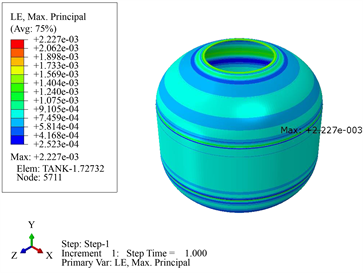(a)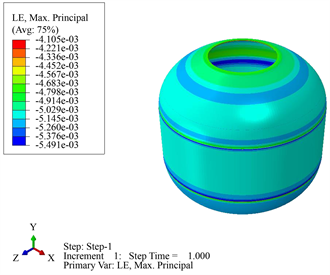(b)

Figure 5. The maximum principal strain cloud diagram of the composite winding layer of the tank. (a) At room temperature; (b) At low temperatures

4.3. 胶层有限元模拟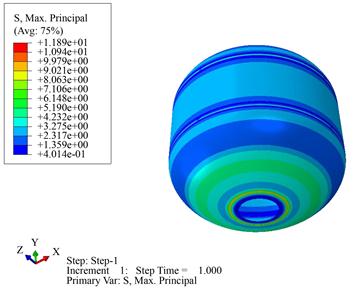(a)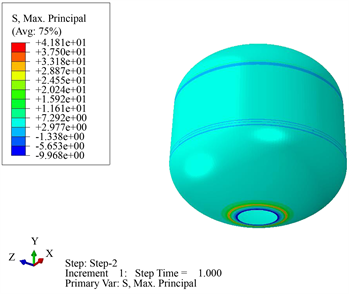(b)

Figure 6. The maximum principal stress cloud of the rubber layer of the tank. (a) At room temperature; (b) At low temperatures

4.4. 法兰连接结构有限元模拟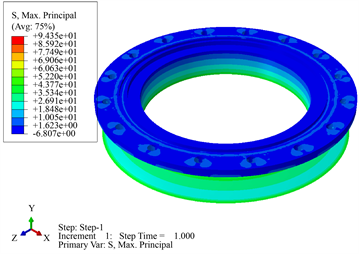(a)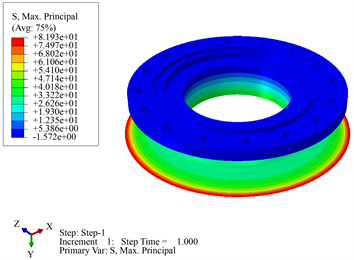(b)

Figure 7. The maximum principal stress cloud of connection structure of storage tank at room temperature. (a) Stress cloud diagram of upper flange; (b) Stress cloud diagram of lower flange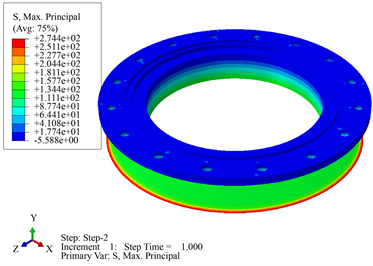(a)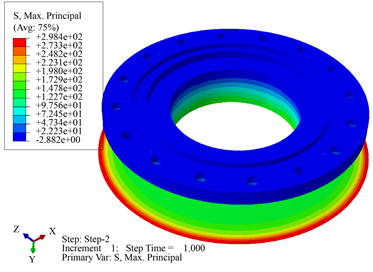(b)

Figure 8. The maximum principal stress cloud of connection structure of storage tank at low temperatures. (a) Stress cloud diagram of upper flange; (b) Stress cloud diagram of lower flange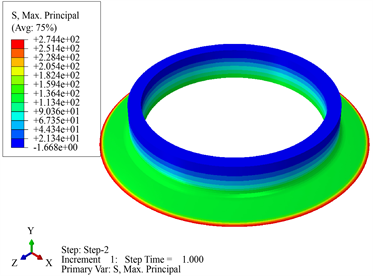(a)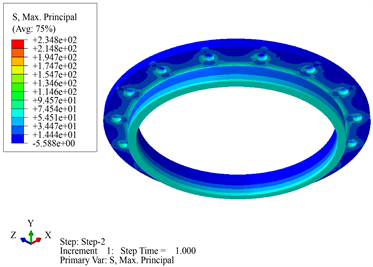(b)

Figure 9. The maximum principal stress cloud of the upper connection of storage tank at low temperatures. (a) Stress cloud of the inner flange; (b) Stress cloud of the outer flange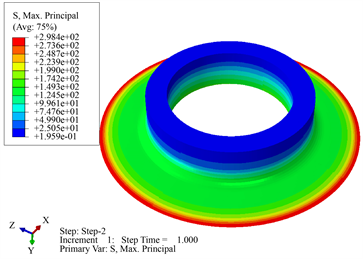(a)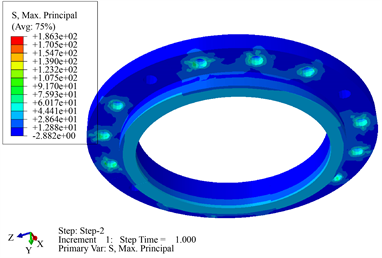(b)

Figure 10. The maximum principal stress cloud of the lower connection of storage tank at low temperatures. (a) Stress cloud of the inner flange; (b) Stress cloud of the outer flange

5. 结论

NOTES

*通讯作者。

  范瑞祥, 容易. 我国新一代中型运载火箭的发展展望[J]. 载人航天, 2013, 19(1): 1-4.  黄诚, 刘德博, 吴会强, 常志龙. 我国航天运载器复合材料贮箱应用展望[J]. 沈阳航空航天大学学报, 2016, 33(2): 27-35.  于建, 晏飞. 可重复使用运载器复合材料低温贮箱应用研究[J]. 火箭推进, 2009, 35(6): 19-22.  Davis, S.E. and Herald, S.D. (2006) Guidelines for the Utilization of Composite Materials in Oxygen Storage Tanks. NASA Marshall Space Night Center.  黄诚, 雷勇军. 大型运载火箭低温复合材料贮箱设计研究进展[J]. 宇航材料工艺, 2015(2): 1-7.  朱平萍, 刘宪力. 大型贮箱结构及工艺性研究[J]. 航天制造技术, 2011(3): 42-45, 58.  辛腾达, 王华, 崔村燕, 等. 贮箱轻量化设计几何参数优化方法[J]. 西安交通大学学报, 2019, 53(7): 153-159.  任明法, 卜璠梓, 吴会强, 等. 飞行器异形复合材料贮箱设计与优化方法研究[J]. 宇航材料工艺, 2014(A01): 31-36.  沈观林, 胡更开. 复合材料力学[M]. 北京: 清华大学出版社, 2006.  施建伟. 基于ABAQUS复合材料层合板渐进损伤有限元分析[D]: [硕士学位论文]. 太原: 中北大学, 2015.  王耀先. 复合材料力学与结构设计[M]. 上海: 华东理工大学出版社, 2012.  Tapeinos, I.G., Koussios, S. and Groves, R.M. (2016) Design and Analysis of a Multi-Cell Subscale Tank for Liquid Hydrogen Storage. International Journal of Hydrogen Energy, 41, 3676-3688.  Ansary, A.M., Damatty, A.A. and Nassef, A.O. (2011) A Couple Finite Element Genetic Algorithm for Optimum Design of Stiffened Liquid-Filled Steel Conical Tanks. Thin-Walled Structures, 49, 482-493.  Fahmy, A.S. and Khalil, A.M. (2016) Wall Thickness Variation Effect on Tank’s Shape Behavior under Critical Harmonic Settlement. Alexandria Engineering Journal, 55, 3205-3209.